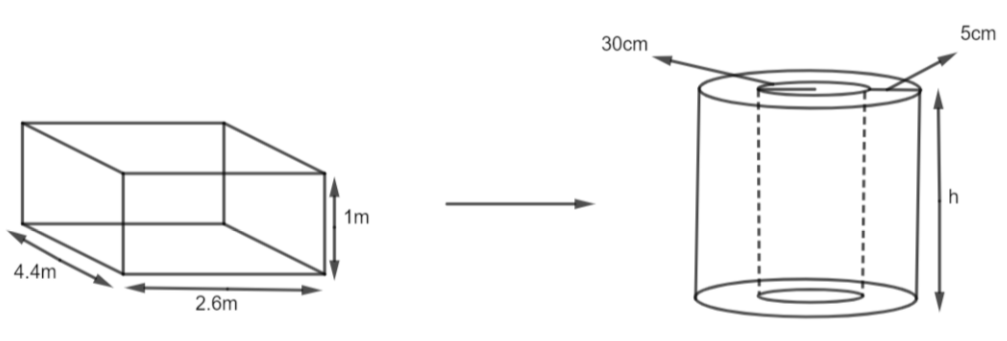Courses
Courses for Kids
Free study material
Free LIVE classes
MoreLIVE
Join Vedantu’s FREE Mastercalss

# A solid iron cuboidal block of dimensions $4.4m\times 2.6m\times 1m$ is recast into a hollow cylindrical pipe of an internal radius 30 cm and thickness 5 cm. Find the length of the pipe.(a) 112 m(b) 110 m(c) 114 m(d) 116 mVerified
334.5k+ views
Hint: The volume of any object before and after the recast always remains the same. So, first, we need to find the volume of the cuboid using the formula $V=L\times B\times H$ and then compare it with the volume of the pipe (Cylinder) which is $V=\pi {{r}^{2}}H$ to find the height of the cylinder.

Complete step-by-step solution:
We have iron cuboidal of dimensions $4.4m\times 2.6m\times 1m.$As we all know that the volume remains fixed, so we can find the volume of the cuboidal block and compare it with the volume of the cylinder. We have the dimensions as $4.4m\times 2.6m\times 1m$
Therefore, the volume of the cuboid is
$V=L\times B\times H$
$\Rightarrow V=4.4\times 2.6\times 1$
$\Rightarrow V=11.44{{m}^{3}}$
So, we get the volume of the cuboidal block as $11.44{{m}^{3}}.$
Now, in the question, we are given that the cylinder is hollow inside. So,
The volume of Cylinder = Volume of Outer Cylindrical part – Volume of Inner Cylindrical part
We have inner radius, r = 30 cm = 0.3 m and thickness, d = 5 cm = 0.05 m.
$R=r+d$
$\Rightarrow R=0.3+0.05$
$\Rightarrow R=0.35m$
Now, we get the volume of the cylinder as
$\text{Volume of cylinder}=\pi {{R}^{2}}H-\pi {{r}^{2}}H$
Here, the height H is the same for the outer and inner parts.
$\Rightarrow \text{Volume of cylinder}=\dfrac{22}{7}\times {{\left( 0.35 \right)}^{2}}\times H-\dfrac{22}{7}{{\left( 0.3 \right)}^{2}}\times H$
$\Rightarrow \text{Volume of cylinder}=\dfrac{22}{7}\left[ {{\left( 0.35 \right)}^{2}}-{{\left( 0.3 \right)}^{2}} \right]H$
Now, to find the height, we will compare the volume of the hollow cylinder with the volume of the cuboidal box. So, we get,
$11.44=\dfrac{22}{7}\left[ {{\left( 0.35 \right)}^{2}}-{{\left( 0.3 \right)}^{2}} \right]H$
$\Rightarrow 11.44=\dfrac{22}{7}\left[ 0.0325 \right]H$
Now, we will solve for H, we get,
$H=\dfrac{11.44\times 7}{22\times 0.0325}$
$\Rightarrow H=\dfrac{80.08}{0.715}$
$\Rightarrow H=112m$
Therefore, we get the height of the cylinder as 112m.
Hence, option (a) is the right answer.

Note: As the cylinder is hollow inside, so we cannot compare the volume of the cuboidal block with the volume of the cylinder with radius r = 0.3m that is, $11.44=\pi \times {{\left( 0.3 \right)}^{2}}\times H,$ this leads us to the wrong answer. For easy calculation of ${{\left( 0.35 \right)}^{2}}-{{0.3}^{2}},$ use ${{a}^{2}}-{{b}^{2}}=\left( a+b \right)\left( a-b \right).$
${{0.35}^{2}}-{{0.3}^{2}}=\left( 0.35+0.3 \right)\left( 0.35-0.3 \right)$
$\Rightarrow {{0.35}^{2}}-{{0.3}^{2}}=0.65\times 0.005$
$\Rightarrow {{0.35}^{2}}-{{0.3}^{2}}=0.0325$

Last updated date: 26th Sep 2023
Total views: 334.5k
Views today: 7.34k